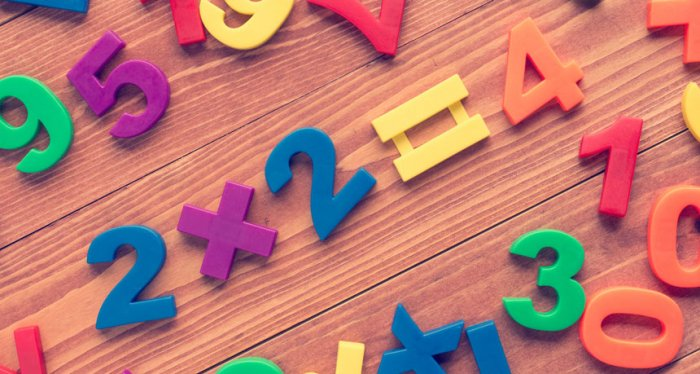### Mathematicians have discovered a new way of multiplying numbers

18 April 2019    Read: 1113Mathematicians have reportedly discovered a new way of multiplying two numbers together.

The new technique is for really large numbers, and if it passes a peer-review, could be the fastest possible way of multiplying whole numbers.

According to the mathematicians, from Australia and France, long multiplicationused to be the only way to multiply - but proved tedious and time-consuming when the numbers became too large.

Even for computers, the long multiplication algorithm, defined as n to the power of, could reportedly take months if each number had a billion digits.

But in a new paper published on the document archive HAL, David Harvey from the University of New South Wales and Joris van der Hoeven of the French national research agency CNRS and École Polytechnique in Palaiseau revealed that a new technique suggested years ago actually works.

The technique, the Schönhage–Strassen algorithm, which “predicted that there should exist an algorithm that multiples n-digit numbers using essentially n*log(n) basic operations,” according to Harvey, was suggested but never proved by German mathematicians.

The new paper proves that the technique is successful in cutting down the time it takes to multiply numbers, with billion-digit numbers theoretically multipled in under 30 seconds using the Schönhage-Strassen algorithm.

“Our paper gives the first known example of an algorithm that achieves this,” Harvey said. “People have been hunting for such an algorithm for almost 50 years. It was not a forgone conclusion that someone would eventually be successful.”

Of the revelation, mathematician and computer scientist Fredrik Johansson from INRIA Bordeaux and Institut de Mathématiques de Bordeaux told New Scientist: "If the result is correct, it's a major achievement in computational complexity theory. The new ideas in this work are likely to inspire further research and could lead to practical improvements down the road."

The Independent

More about: